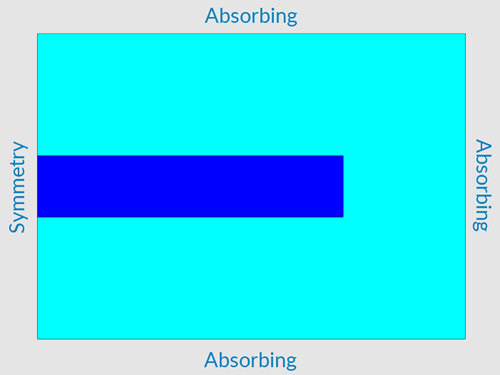# Setting the Domain Boundaries

We need to set up the external boundaries of our model to reflect how the areas beyond the model would behave in the real world.

By default all domain boundaries are set to Free, which means nodes can move freely with no external forces acting upon them. You would use this if the edge of a model was at an air boundary, where the movement of boundary notes is unrestricted.

In our model, we'll change the X Minimum domain boundary to Symmetry since, remember, our model is axi-symmetrical around the Y axis. We'll change the other three domain boundaries to Absorbing, which means that energy continues past the boundary with no reflections.## Setting the Boundaries

To set the domain boundaries, follow these steps:

1. In the Model Tree, click Domain Boundaries.
2. In the Properties window, expand X Minimum and, for Boundary Type, select Symmetry.
3. Expand X Maximum, Y Minimum and Y Maximum and, for each, select Absorbing for Boundary Type.

## Setting the Simulation Run Time

Before we move on, let's quickly set the simulation run time. As the name suggests, this defines how long the model runs for. It's measured in seconds.

1. In the Model Tree, click Analysis.
2. In the Properties window, for Simulation Run Time (s), type 5e-5.

## What Next?

Next we'll add the outputs that we want from the simulation.